Numbers, numbers, numbers everywhere!

# Pre-Algebra - Simplifying and Multiplying Radicals (Part 1)

This Math quiz is called 'Pre-Algebra - Simplifying and Multiplying Radicals (Part 1)' and it has been written by teachers to help you if you are studying the subject at middle school. Playing educational quizzes is a fabulous way to learn if you are in the 6th, 7th or 8th grade - aged 11 to 14.

It costs only \$12.50 per month to play this quiz and over 3,500 others that help you with your school work. You can subscribe on the page at Join Us

As you have previously learned, a radical is simply an expression that uses a root such as the square root of or the cube root of. Often, you will be asked to simplify by multiplying a “radical expression.” To understand what this means, let’s look at the following problem using the variable “x”. We will be looking for the simplified solution using multiplication. (See the example below.)

Go straight to Quiz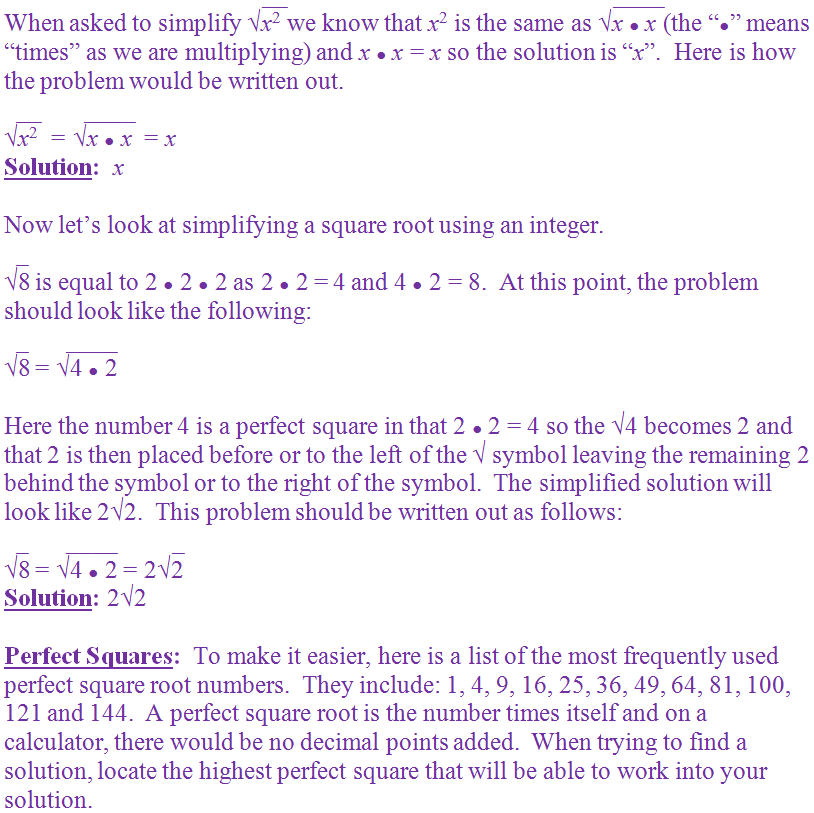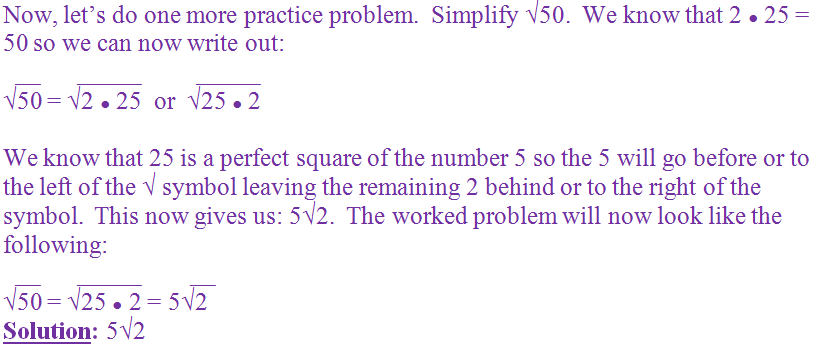Question 1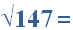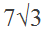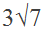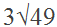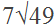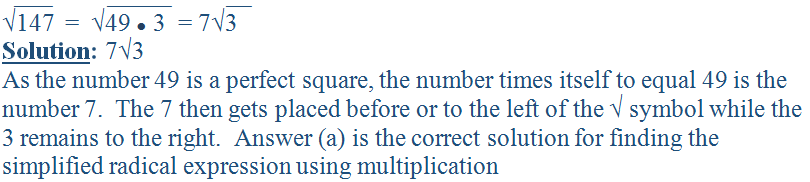Question 2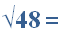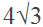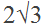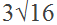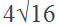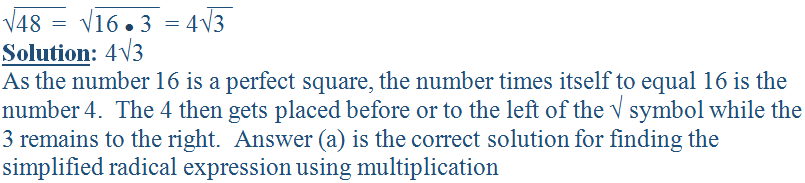Question 3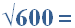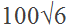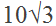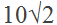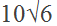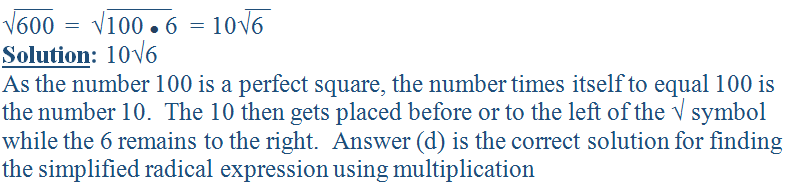Question 4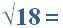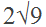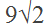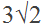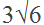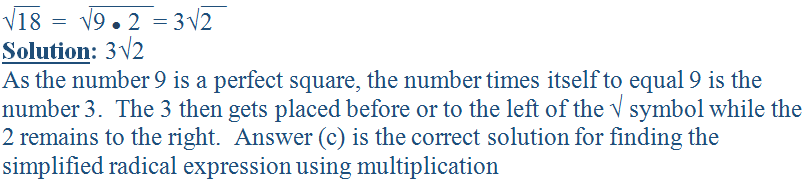Question 5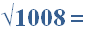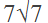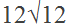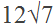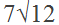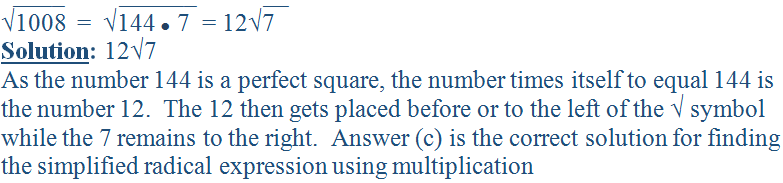Question 6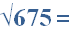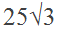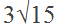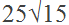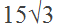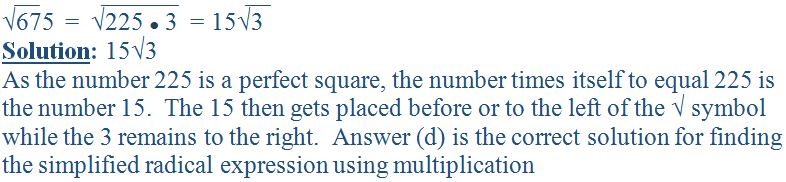Question 7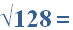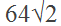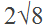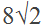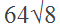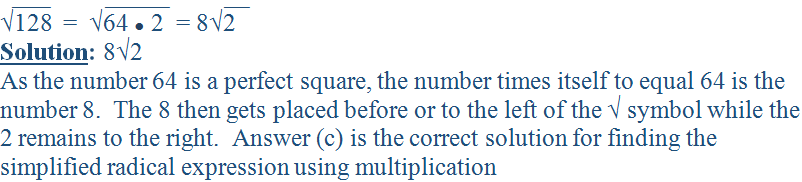Question 8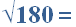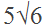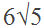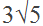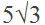Question 9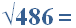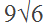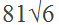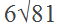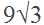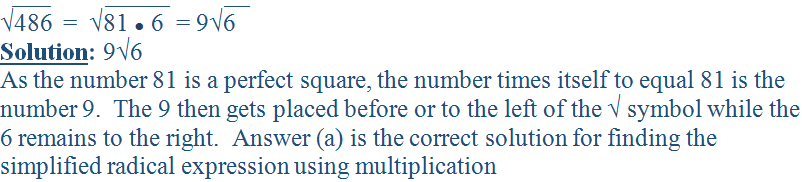Question 10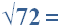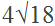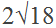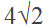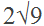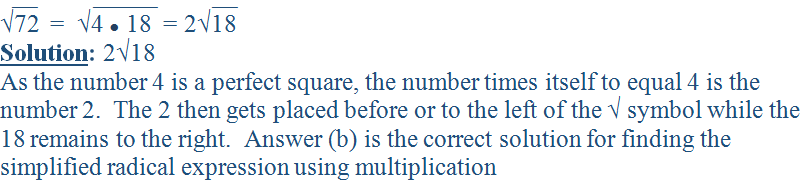Author:  Christine G. Broome